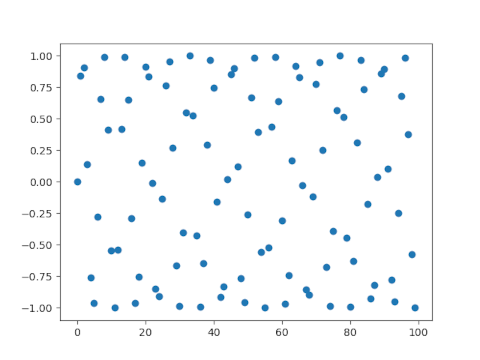# Sine of integers

The sine function has period 2π, an irrational number. and so if we take the sines of the integers, we’re going to get a somewhat random sequence. (I’m assuming, as always that we’re working in radians. The sines of integer numbers of degrees are much less interesting.)

Here’s a plot of the sines of 0, 1, 2, …, 99.The points are not literally random, but they’re what Marsaglia would call higgledy-piggledy .

Since the points are higgledy-piggledy, it doesn’t seem possible that we could find a lower bound on |sin(n)|, but there is one. For all positive integers n,

|sin(n)| > 2n.

This result may be due to Christopher Stuart, but in any case he proved  a stronger version of this theorem, showing that the 2 on the right hand side can be replaced with any number

α > (sin(3))-1/3 = 1.9207….

## Related posts

 “If the numbers are not random, they are at least higgledy-piggledy.” — RNG researcher George Marsaglia

 Christopher Stuart. An Inequality Involving sin(n). The American Mathematical Monthly , Feb 2018, Vol. 125, No. 2, pp. 173–174

## 2 thoughts on “Sine of integers”

1. I remember an Analysis homework problem that asked us to prove {sin(n)} was dense in [-1-,1]

2. Right. So sin(n) gets arbitrarily close to zero, but the smaller your epsilon is the longer you have to wait.